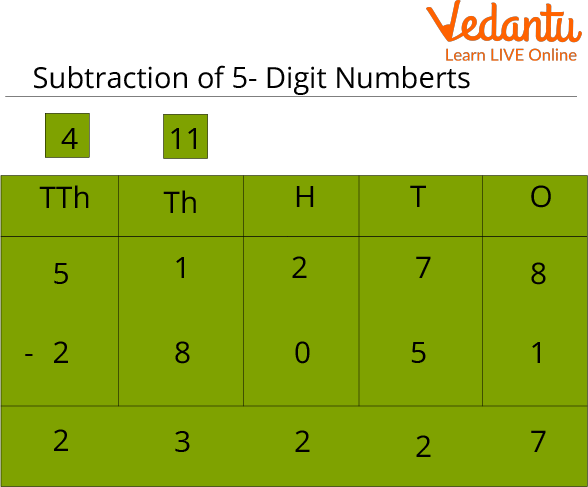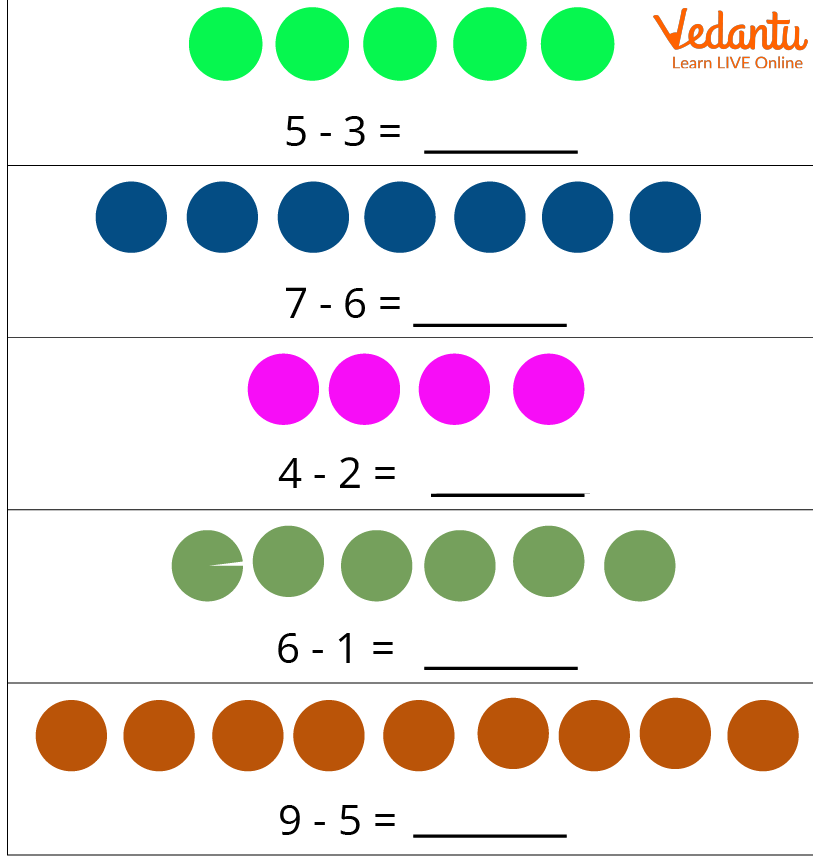Courses
Courses for Kids
Free study material
Free LIVE classes
More

# Properties of Subtraction## Understanding the Properties of Subtraction

According to the algebraic concept known as the "subtraction property of equality," if a value is subtracted from two equal quantities, the resulting differences are also equal.

The subtraction property of equality states that in order to maintain equality if we take a number away from one side of equality, we must also take that same amount away from the other side.

Comparable to the equality's addition property is this property. The properties of equality range from the addition property to the division property to the reflexive property to the multiplication property, among others. By applying the identical mathematical operation of subtraction to both sides of an equality, the subtraction property of equality seeks to balance an equation.

Let's look at an illustration to comprehend this better. There are two bags with ten balls each. Now, if we remove three balls from bag 1, we must remove three from bag 2 to maintain the same number of balls in bags 1 and 2. Thus, after releasing three balls from each bag, there are seven balls left in each bag.Subtraction of 5-Digit Number

## Properties of Whole-Number Subtraction

Property 1:

If two whole numbers a and b are such that A > B or A = B, then A - B is also a whole number. If A < B, then it is impossible to subtract B from A in whole numbers.

For instance,

10 - 5 = 5

23 - 12 = 11

100 - 99 = 1

Property 2: Commutative Property of Subtraction

Whole numbers cannot be subtracted cumulatively, hence even if a and b are both whole numbers, a - b does not always equal (B - A).

Verification:

We are aware that 9 – 5 = 4, however, 5 – 9 is illogical. Additionally, 125 - 75 = 50, but 75 - 125 is impractical. In other words, if A > B, then A - B is a whole number but B - A is not feasible, and if B > A, then B - A is a whole number but A - B is not possible for two whole numbers A and B. This is the Commutative property of subtraction.

Therefore, (A -B) is generally not equal to (B - A).

Property 3:

A - 0 equals A if a is any whole number other than zero, but 0 - A is not defined.

Verification:

Although 0 - 15 is not feasible, we know that 15 - 0 equals 15.

Property 4: Associative Property of Subtraction

Whole number subtraction is not associative. In other words, if A, B, and C are three whole numbers, A - (B - C) is generally not equivalent to (A - B) - C. This is the Associative Property of Subtraction.

Verification:

Associative Property of Subtraction Example

10 - (9 - 3) = 10 - 6 = 4,

thus, (10 - 9) - 3 = 1 - 3 = - 2

Now because of that 10 - (9 - 3) (10 - 9) - 3.

## Zero Property of Subtraction

When a number is subtracted from zero, the result is the original number.

For instance,

(i) 5645 - 0 = 5645;

(ii) 987 - 0 = 987

## Properties of a Number Subtracted from Itself

The difference is zero when a number is subtracted from itself, according to the properties of subtraction.

For instance,

323 - 323 = 0

12 - 12 =0

## Properties of Subtraction Worksheet

Children can practise from properties of subtraction worksheets.Properties of Subtraction Worksheet

## Summary

A mathematical process called subtraction involves taking a portion of one value and subtracting it from another. You subtract two numbers when you do subtraction. The minuend is the initial digit in a subtraction equation. This is the figure from which we deduct. The subtraction is the name of the second number. We take away this from the minute. When we subtract, the result is the difference.

Last updated date: 20th Sep 2023
Total views: 116.7k
Views today: 2.16k

## FAQs on Properties of Subtraction

1. What is subtracting?

To subtract in mathematics is to take something away from a group or a number of objects. The group's total number of items decreases or becomes lower when we subtract from it. The components of a subtraction issue are the minuend, subtrahend, and difference. The number 7 is the minuend, the number 3 is the subtrahend, and the number 4 is the difference in the subtraction problem, 7 - 3 = 4.

Another example is if a class has 16 students, 9 of them are girls, we can determine the number of boys in the class by deducting 9 from 16. (16 - 9 = 7). There are 7 boys in the class, as far as we know.

2. What kinds of subtraction are there?

The numerous techniques employed in subtraction are referred to as the forms of subtraction. Examples include subtracting small amounts with and without regrouping, subtracting using number lines and charts, and subtracting using your fingers. There are various ways to learn subtraction, including subtraction tactics. For instance, splitting the Tens and Ones and then subtracting them separately, utilising a number line, using a place value chart, and many others.

Subtraction can be applied in a variety of real-world situations. Assume you had five apples and your friend had three. We may calculate the number of remaining apples by subtracting: 5 - 3 Equals 2. Therefore, you are left with 2 apples.

3. What is subtraction property order?

This means that we cannot group any two whole numbers and subtract them first. Order of subtraction is an important factor. If 'a', 'b', and 'c' are the three whole numbers, then a − (b − c) ≠ (a − b) − c.